# mne.time_frequency.tfr_morlet#

mne.time_frequency.tfr_morlet(inst, freqs, n_cycles, use_fft=False, return_itc=True, decim=1, n_jobs=None, picks=None, zero_mean=True, average=True, output='power', verbose=None)[source]#

Compute Time-Frequency Representation (TFR) using Morlet wavelets.

Same computation as tfr_array_morlet, but operates on Epochs or Evoked objects instead of NumPy arrays.

Parameters:
inst

The epochs or evoked object.

freqsarray of float, shape (n_freqs,)

The frequencies of interest in Hz.

n_cyclesint | array of int, shape (n_freqs,)

Number of cycles in the wavelet, either a fixed number or one per frequency. The number of cycles n_cycles and the frequencies of interest freqs define the temporal window length. See notes for additional information about the relationship between those arguments and about time and frequency smoothing.

use_fftbool, default False

The fft based convolution or not.

return_itcbool, default True

Return inter-trial coherence (ITC) as well as averaged power. Must be False for evoked data.

decimint | slice, default 1

To reduce memory usage, decimation factor after time-frequency decomposition.

Note

Decimation is done after convolutions and may create aliasing artifacts.

n_jobs

The number of jobs to run in parallel. If -1, it is set to the number of CPU cores. Requires the joblib package. None (default) is a marker for ‘unset’ that will be interpreted as n_jobs=1 (sequential execution) unless the call is performed under a joblib.parallel_backend() context manager that sets another value for n_jobs.

picks

The indices of the channels to decompose. If None, all available good data channels are decomposed.

zero_meanbool, default True

Make sure the wavelet has a mean of zero.

New in version 0.13.0.

averagebool, default True

If False return an EpochsTFR containing separate TFRs for each epoch. If True return an AverageTFR containing the average of all TFRs across epochs.

Note

Using average=True is functionally equivalent to using average=False followed by EpochsTFR.average(), but is more memory efficient.

New in version 0.13.0.

outputstr

Can be "power" (default) or "complex". If "complex", then average must be False.

New in version 0.15.0.

verbose

Control verbosity of the logging output. If None, use the default verbosity level. See the logging documentation and mne.verbose() for details. Should only be passed as a keyword argument.

Returns:
power

The averaged or single-trial power.

itc

The inter-trial coherence (ITC). Only returned if return_itc is True.

Notes

The Morlet wavelets follow the formulation in .

In spectrotemporal analysis (as with traditional fourier methods), the temporal and spectral resolution are interrelated: longer temporal windows allow more precise frequency estimates; shorter temporal windows “smear” frequency estimates while providing more precise timing information.

Time-frequency representations are computed using a sliding temporal window. Either the temporal window has a fixed length independent of frequency, or the temporal window decreases in length with increased frequency.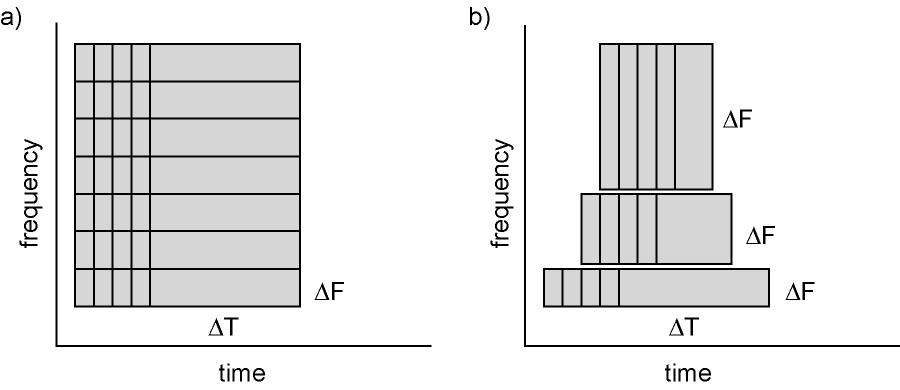Figure: Time and frequency smoothing. (a) For a fixed length temporal window the time and frequency smoothing remains fixed. (b) For temporal windows that decrease with frequency, the temporal smoothing decreases and the frequency smoothing increases with frequency. Source: FieldTrip tutorial: Time-frequency analysis using Hanning window, multitapers and wavelets.

In MNE-Python, the temporal window length is defined by the arguments freqs and n_cycles, respectively defining the frequencies of interest and the number of cycles: $$T = \frac{\mathtt{n\_cycles}}{\mathtt{freqs}}$$

A fixed number of cycles for all frequencies will yield a temporal window which decreases with frequency. For example, freqs=np.arange(1, 6, 2) and n_cycles=2 yields T=array([2., 0.7, 0.4]).

To use a temporal window with fixed length, the number of cycles has to be defined based on the frequency. For example, freqs=np.arange(1, 6, 2) and n_cycles=freqs / 2 yields T=array([0.5, 0.5, 0.5]).

In wavelet analysis, the oscillation that is defined by n_cycles is tapered by a Gaussian taper, i.e., the edges of the wavelet are dampened. This means that reporting the number of cycles is not necessarily helpful for understanding the amount of temporal smoothing that has been applied (see ). Instead, the full width at half-maximum (FWHM) of the wavelet can be reported.

The FWHM of the wavelet at a specific frequency is defined as: $$\mathrm{FWHM} = \frac{\mathtt{n\_cycles} \times \sqrt{2 \ln{2}}}{\pi \times \mathtt{freq}}$$ (cf. eq. 4 in ).

See mne.time_frequency.morlet() for more information about the Morlet wavelet.

References

## Examples using mne.time_frequency.tfr_morlet#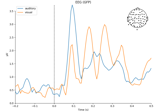Overview of MEG/EEG analysis with MNE-Python

Overview of MEG/EEG analysis with MNE-Python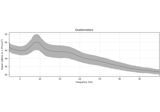Frequency and time-frequency sensor analysis

Frequency and time-frequency sensor analysis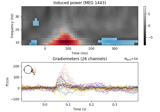Non-parametric 1 sample cluster statistic on single trial power

Non-parametric 1 sample cluster statistic on single trial power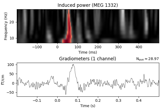Non-parametric between conditions cluster statistic on single trial power

Non-parametric between conditions cluster statistic on single trial power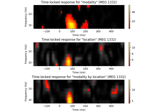Mass-univariate twoway repeated measures ANOVA on single trial power

Mass-univariate twoway repeated measures ANOVA on single trial power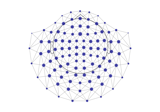Spatiotemporal permutation F-test on full sensor data

Spatiotemporal permutation F-test on full sensor data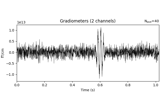Time-frequency on simulated data (Multitaper vs. Morlet vs. Stockwell vs. Hilbert)

Time-frequency on simulated data (Multitaper vs. Morlet vs. Stockwell vs. Hilbert)Compute source level time-frequency timecourses using a DICS beamformer

Compute source level time-frequency timecourses using a DICS beamformer